## "A mixture of date references caused missing values to be returned for a period calculation"

Version SAS Visual Analytics 8.5.2

I think this is for sure a bug in Visual Analytics.

I've tried without success with any possible date formats, changing from "inferred" to "byMonth" and playing with the filter options.

Nothing. It fails.

It doesn't matter if I create the date from sas code beforehand or use the DatefromMdy as created data item.

And it fails with a simple table having only few rows and no distinct class crossings.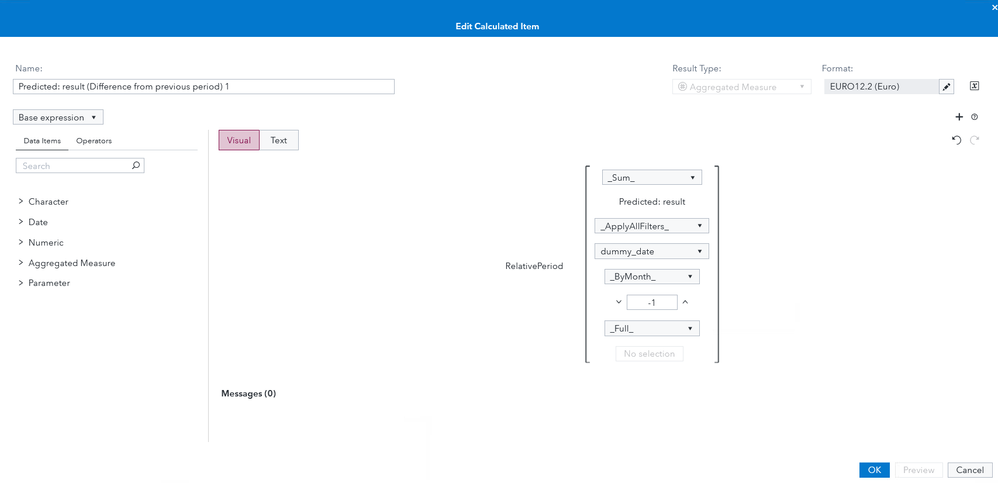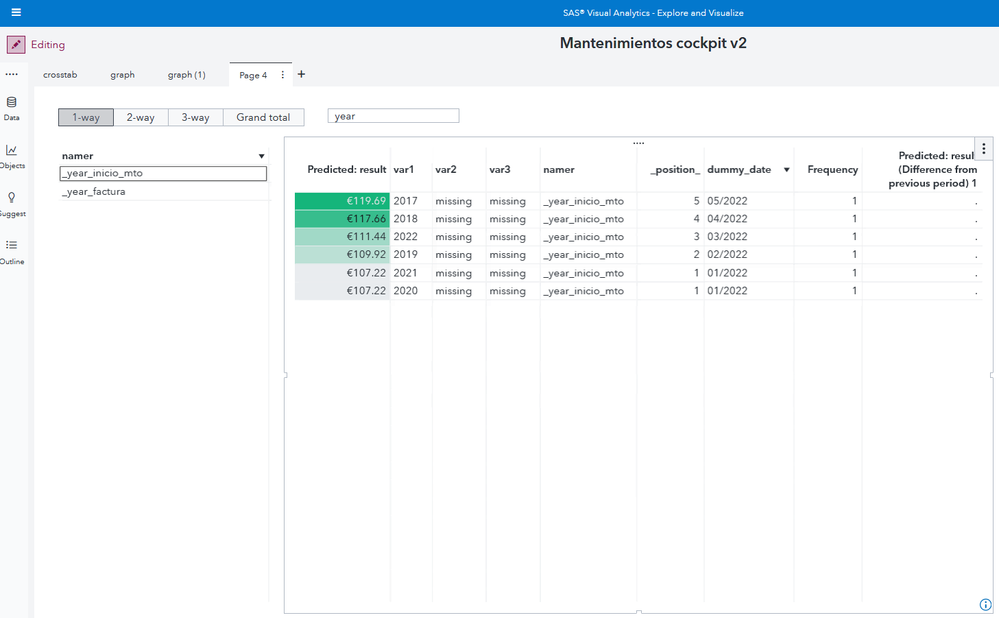1 ACCEPTED SOLUTION

Accepted Solutions

## Re: "A mixture of date references caused missing values to be returned for a period calculation

Thanks! That's what I was hoping to see and you may be right, there might be a bug here since of the data used in your list table, dummy_date is clearly the only date type. Generally speaking this should work though and I can't tell if it's specific to your data or what. A quick way to rule that out is if you can get to the Sample CAS tables, Warranty_Claims_0117 has Date data items in it. You could load that table into a Visual Analytics report and do this:

1. Change the format of Ship Date to MMMYYYY

2. Create this calc item:
RelativePeriod(_Sum_, 'Usage Value'n, _IgnoreAllTimeFrameFilters_, 'Ship Date'n, _Inferred_, -1, _Full_, {Date})
3. Add Ship Date, Usage Value, and this new calc item to a list table.

It would be helpful to know if RelativePeriod works in general for you. Then we might be able to reasonably suspect your data itself although for the moment I can't imagine why it would be.

Assuming we can confirm that, I think you might need to open a tech support track to debug this further.

7 REPLIES 7

## Re: "A mixture of date references caused missing values to be returned for a period calculation

That warning means your table has more than one Date with different formats referenced, and the periodic calculation will not work that way. I would expect it to work if your list table just had dummy_date and the "Predicted: result (Difference from previous period)" calculation in it.

But if any of those other data items are dates of another format (for instance is var1 a datetype with format of Year?) then you'll have this problem.

If you're not sure, one we can check for you is if you click the list table object and press Ctrl+Alt+Q on your keyboard. A new window will appear for Query Diagnostics. Save what you see in the Query tab and attach that to this post, and we'll see what's going on in it.

## Re: "A mixture of date references caused missing values to be returned for a period calculation

<?xml version="1.0" encoding="UTF-8"?>
<SASReport xmlns="http://www.sas.com/sasreportmodel/bird-4.2.4" createdLocale="en" createdVersion="4.2.4" dateCreated="2022-10-26T14:56:05Z" dateModified="2022-10-26T14:56:05Z" nextUniqueNameIndex="1541">
<RelationalDataItem name="bi1309" base="bi1214"/>
<RelationalDataItem name="bi1317" base="bi1221"/>
<RelationalDataItem name="bi1345" base="bi1344"/>
<RelationalDataItem name="bi1348" base="bi1346"/>
<RelationalDataItem name="bi1354" base="bi1347"/>
<RelationalDataItem name="bi1365" base="bi1362"/>
<RelationalDataItem name="bi1518" base="bi1364"/>
<RelationalDataItem name="bi1523" base="bi1243"/>
<RelationalDataItem name="bi1540" base="bi1539"/>
<RelationalQuery detail="false">
<Axes>
<Axis type="column">
</Axis>
</Axes>
<SortItems>
<SortItem ref="bi1518" sortDirection="descending"/>
</SortItems>
</RelationalQuery>
<ResultDefinitions>
<ResultDefinition purpose="primary" maxRowsLookup="listTable" maxRowsBehavior="truncate" name="dd1316"/>
</ResultDefinitions>
<AppliedFilters/>
<DataSources>
<DataSource label="MTOS_CRISTAL_CLEAR2" type="relational" name="ds1177">
<CasResource server="cas-shared-default" library="Public" table="MTOS_CRISTAL_CLEAR2" locale="en_US"/>
<DataItem name="bi1214" xref="namer"/>
<DataItem name="bi1221" xref="P_result" format="EURO12.2" aggregation="average"/>
<CalculatedItem dataType="string" name="bi1344" label="var1" usage="categorical" format="\$." aggregation="sum">
<Expression>cond(in(\${bi1329,binned},'_deviation_kms'),\${bi1343,binned},cond(in(\${bi1329,binned},'_KMJECONT'),\${bi1182,binned},cond(in(\${bi1329,binned},'_year_factura'),\${bi1184,binned},cond(in(\${bi1329,binned},'_year_inicio_mto'),\${bi1185,binned},cond(in(\${bi1329,binned},'canal'),\${bi1189,binned},cond(in(\${bi1329,binned},'custom_wear_and_tear'),\${bi1192,binned},cond(in(\${bi1329,binned},'dealer_name'),\${bi1193,binned},cond(in(\${bi1329,binned},'flag_early_ld'),\${bi1198,binned},cond(in(\${bi1329,binned},'from_brand'),\${bi1199,binned},cond(in(\${bi1329,binned},'from_gama_agg'),\${bi1200,binned},cond(in(\${bi1329,binned},'MODSERMT'),\${bi1212,binned},cond(in(\${bi1329,binned},'situation'),\${bi1232,binned},cond(in(\${bi1329,binned},'skipped_longdrive'),\${bi1233,binned},'missing')))))))))))))</Expression>
</CalculatedItem>
<CalculatedItem dataType="string" name="bi1329" label="parse1" usage="categorical" format="\$." aggregation="sum">
<Expression>getWordAtTokenIndex(\${bi1214,binned},1)</Expression>
</CalculatedItem>
<CalculatedItem dataType="string" name="bi1343" label="deviation_kms (char)" usage="categorical" format="\$." aggregation="sum">
<Expression>applySASFormat(\${bi1180,raw},\$SASFormat{BEST12.})</Expression>
</CalculatedItem>
<DataItem name="bi1180" xref="_deviation_kms"/>
<DataItem name="bi1182" xref="_KMJECONT"/>
<DataItem name="bi1184" xref="_year_factura"/>
<DataItem name="bi1185" xref="_year_inicio_mto"/>
<DataItem name="bi1189" xref="canal"/>
<DataItem name="bi1192" xref="custom_wear_and_tear"/>
<DataItem name="bi1193" xref="dealer_name"/>
<DataItem name="bi1198" xref="flag_early_ld"/>
<DataItem name="bi1199" xref="from_brand"/>
<DataItem name="bi1200" xref="from_gama_agg"/>
<DataItem name="bi1212" xref="MODSERMT"/>
<DataItem name="bi1232" xref="situation"/>
<DataItem name="bi1233" xref="skipped_longdrive"/>
<CalculatedItem dataType="string" name="bi1346" label="var2" usage="categorical" format="\$." aggregation="sum">
<Expression>cond(in(\${bi1330,binned},'_deviation_kms'),\${bi1343,binned},cond(in(\${bi1330,binned},'_KMJECONT'),\${bi1182,binned},cond(in(\${bi1330,binned},'_year_factura'),\${bi1184,binned},cond(in(\${bi1330,binned},'_year_inicio_mto'),\${bi1185,binned},cond(in(\${bi1330,binned},'canal'),\${bi1189,binned},cond(in(\${bi1330,binned},'custom_wear_and_tear'),\${bi1192,binned},cond(in(\${bi1330,binned},'dealer_name'),\${bi1193,binned},cond(in(\${bi1330,binned},'flag_early_ld'),\${bi1198,binned},cond(in(\${bi1330,binned},'from_brand'),\${bi1199,binned},cond(in(\${bi1330,binned},'from_gama_agg'),\${bi1200,binned},cond(in(\${bi1330,binned},'MODSERMT'),\${bi1212,binned},cond(in(\${bi1330,binned},'situation'),\${bi1232,binned},cond(in(\${bi1330,binned},'skipped_longdrive'),\${bi1233,binned},'missing')))))))))))))</Expression>
</CalculatedItem>
<CalculatedItem dataType="string" name="bi1330" label="parse2" usage="categorical" format="\$." aggregation="sum">
<Expression>cond(gt(\${bi1245,raw},1),getWordAtTokenIndex(substring(\${bi1214,binned},plus(find(\${bi1214,binned},' x '),3),length(\${bi1214,binned})),1),' ')</Expression>
</CalculatedItem>
<DataItem name="bi1245" xref="ways_helper"/>
<CalculatedItem dataType="string" name="bi1347" label="var3" usage="categorical" format="\$." aggregation="sum">
<Expression>cond(in(\${bi1342,binned},'_deviation_kms'),\${bi1343,binned},cond(in(\${bi1342,binned},'_KMJECONT'),\${bi1182,binned},cond(in(\${bi1342,binned},'_year_factura'),\${bi1184,binned},cond(in(\${bi1342,binned},'_year_inicio_mto'),\${bi1185,binned},cond(in(\${bi1342,binned},'canal'),\${bi1189,binned},cond(in(\${bi1342,binned},'custom_wear_and_tear'),\${bi1192,binned},cond(in(\${bi1342,binned},'dealer_name'),\${bi1193,binned},cond(in(\${bi1342,binned},'flag_early_ld'),\${bi1198,binned},cond(in(\${bi1342,binned},'from_brand'),\${bi1199,binned},cond(in(\${bi1342,binned},'from_gama_agg'),\${bi1200,binned},cond(in(\${bi1342,binned},'MODSERMT'),\${bi1212,binned},cond(in(\${bi1342,binned},'situation'),\${bi1232,binned},cond(in(\${bi1342,binned},'skipped_longdrive'),\${bi1233,binned},'missing')))))))))))))</Expression>
</CalculatedItem>
<CalculatedItem dataType="string" name="bi1342" label="parse3" usage="categorical" format="\$." aggregation="sum">
<Expression>cond(eq(\${bi1245,raw},3),getWordAtTokenIndex(substring(\${bi1214,binned},minus(length(\${bi1214,binned}),plus(find(reverse(\${bi1214,binned}),' x '),-2)),length(\${bi1214,binned})),1),' ')</Expression>
</CalculatedItem>
<DataItem name="bi1362" xref="_position_"/>
<CalculatedItem dataType="date" name="bi1364" label="dummy_date" usage="categorical" format="MMYY8" aggregation="sum">
<Expression>mdy(cond(eq(mod(\${bi1362,raw},12),0),12,mod(\${bi1362,raw},12)),1,plus(2022,floor(div(\${bi1362,raw},12))))</Expression>
</CalculatedItem>
<PredefinedDataItem calculation="totalCount" name="bi1243" label="Frequency" usage="quantitative" format="COMMA12."/>
<AggregateCalculatedItem dataType="double" name="bi1539" label="Predicted: result (Difference from previous period) 1" format="EURO12.2">
<Expression>relativePeriod(sum,\${bi1221,raw},allFiltersApply,\${bi1364,raw},inferred,-1,full)</Expression>
</AggregateCalculatedItem>
<DataItem name="bi1246" xref="ways_info"/>
</DataSource>
</DataSources>
<History>
<Versions>
<Version key="4.2.4" lastDate="2022-10-26T14:56:05Z"/>
</Versions>
</History>
<SASReportState date="2022-10-26T14:56:05Z">
<Parameters>
<Parameter prompt="pr588" dataType="double">10000</Parameter>
<Parameter prompt="pr1326" dataType="string">'year'</Parameter>
</Parameters>
<Data>
<queryRequests>
<queryRequest data="dd1314" dataLevel="custom">
<expressions>
<expression type="filter" dataStage="detail">eq(\${bi1246},'1-way')</expression>
<expression type="filter" dataStage="detail">eq(\${bi1214},'_year_inicio_mto')</expression>
</expressions>
</queryRequest>
</queryRequests>
</Data>
</SASReportState>
</SASReport>

## Re: "A mixture of date references caused missing values to be returned for a period calculation

Well unfortunately this isn't showing me the types and formats of all of the data items like I was hoping it would, so let's try a different approach. If you go to create a new calculated item, in the Data Items pane it should group all Dates or Date types together, can you show me what it has? Like this: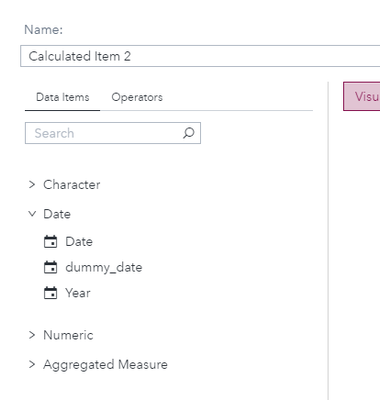Also did you try the list table with just the two data items dummy_date and "Predicted: result (Difference from previous period) 1"? Did that work or do you see the same problem/warning?

## Re: "A mixture of date references caused missing values to be returned for a period calculation

No, reducing the list table to to date and periodic aggregated measure does not solve the problem.

I use this fake_date (created by code in sas studio) and dummy_date as means for having access to the aggregated periodic measures.

I order the data by predicted result (grouped by namer) and derive a position.

the position variable is used to construct the dates (intnx vía code) and screenshot as data item.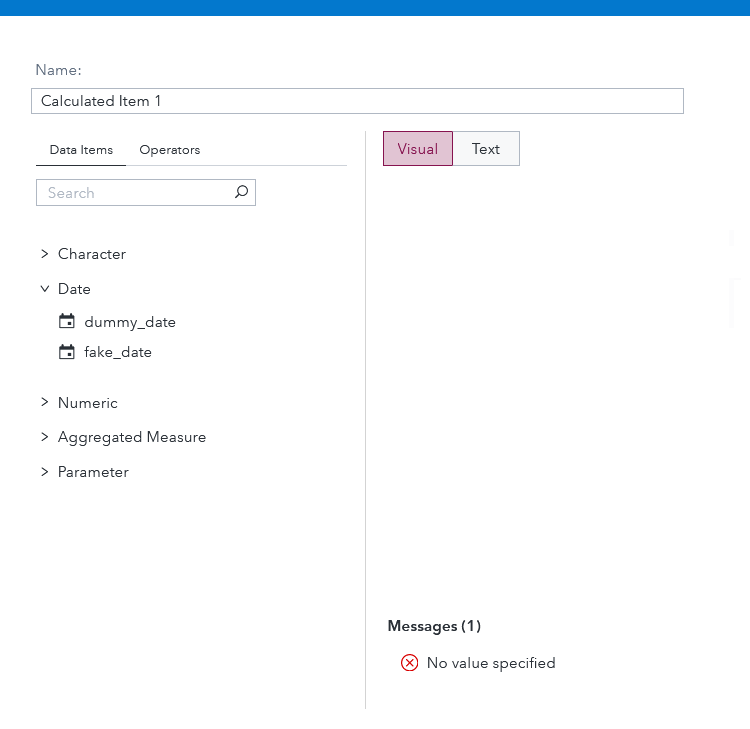## Re: "A mixture of date references caused missing values to be returned for a period calculation

Thanks! That's what I was hoping to see and you may be right, there might be a bug here since of the data used in your list table, dummy_date is clearly the only date type. Generally speaking this should work though and I can't tell if it's specific to your data or what. A quick way to rule that out is if you can get to the Sample CAS tables, Warranty_Claims_0117 has Date data items in it. You could load that table into a Visual Analytics report and do this:

1. Change the format of Ship Date to MMMYYYY

2. Create this calc item:
RelativePeriod(_Sum_, 'Usage Value'n, _IgnoreAllTimeFrameFilters_, 'Ship Date'n, _Inferred_, -1, _Full_, {Date})
3. Add Ship Date, Usage Value, and this new calc item to a list table.

It would be helpful to know if RelativePeriod works in general for you. Then we might be able to reasonably suspect your data itself although for the moment I can't imagine why it would be.

Assuming we can confirm that, I think you might need to open a tech support track to debug this further.

## Re: "A mixture of date references caused missing values to be returned for a period calculation

My frequency is 1 for some of the rows, so if predicted result is unique it gets its own position, there are no ties.

Anf my dates are perfect, meaning they are end-aligned for each month.

I see these diferences.

I even tried my report with an aggregated table, but this doesn't fix it either.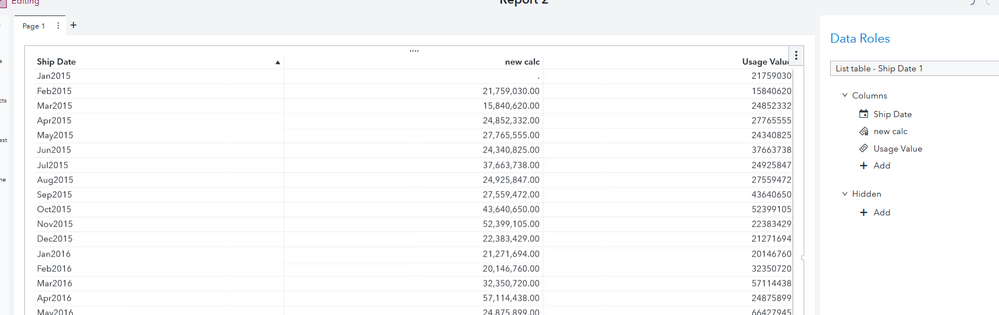## Re: "A mixture of date references caused missing values to be returned for a period calculation

Finally it works!!!

and it seems that date has to be in mmmyyyy format.

It fails the date variable is not unique.

In my case, year '2020' and '2021' share the same predicted result --> same position --> same date var.

So I have to aggregate and then it works.

Thank yo very much.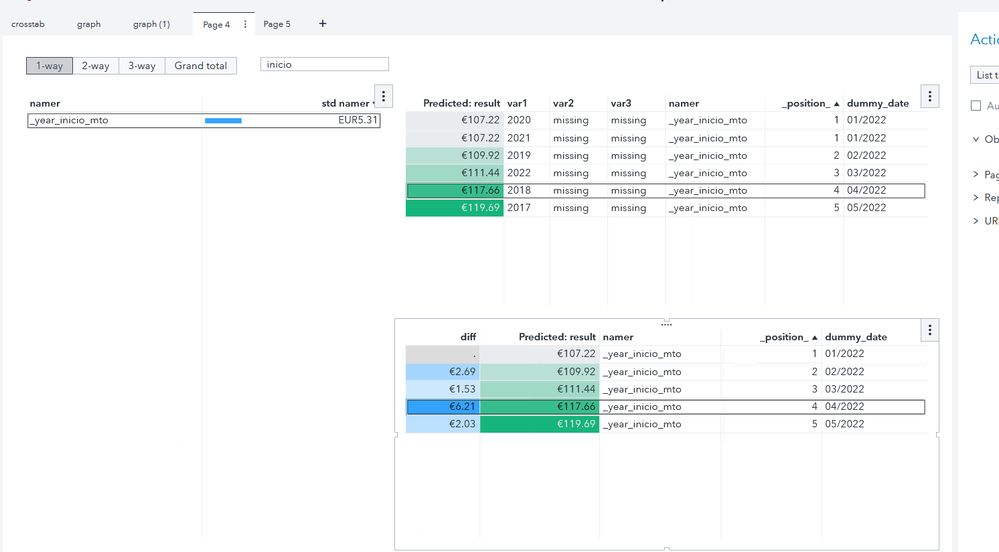Discussion stats
• 7 replies
• 441 views
• 0 likes
• 2 in conversation